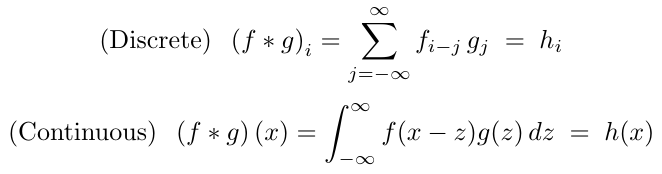# Fourier Convolutions in PyTorchMath and code for efficiently computing large convolutions with FFTs.

ConvolutionsConvolutions are ubiquitous in data analysis. For decades, they’ve been used in signal and image processing. More recently, they became an important ingredient in modern neural networks. You’ve probably encountered convolutions if you work with data at all.Mathematically, convolutions are expressed as:Although discrete convolutions are more common in computational applications, I’ll be working with the continuous form for most of this article, because it is much easier to prove the Convolution Theorem (discussed below) using continuous variables. After that, we will return to the discrete case and implement it in PyTorch using Fourier transforms. Discrete convolutions can be viewed as an approximation of continuous ones, where continuous functions are discretized on a regular grid. So we will not re-prove the Convolution Theorem for the discrete case.

## Hire Machine Learning Developers in India

We supply you with world class machine learning experts / ML Developers with years of domain experience who can add more value to your business.

## How To Plot A Decision Boundary For Machine Learning Algorithms in Python

How To Plot A Decision Boundary For Machine Learning Algorithms in Python, you will discover how to plot a decision surface for a classification machine learning algorithm.

## How To Get Started With Machine Learning With The Right Mindset

You got intrigued by the machine learning world and wanted to get started as soon as possible, read all the articles, watched all the videos, but still isn’t sure about where to start, welcome to the club.

## What is Supervised Machine Learning

What is neuron analysis of a machine? Learn machine learning by designing Robotics algorithm. Click here for best machine learning course models with AI

## Python For Machine Learning | Machine Learning With Python

Python For Machine Learning | Machine Learning With Python, you will be working on an end-to-end case study to understand different stages in the Machine Learning (ML) life cycle. This will deal with 'data manipulation' with pandas and 'data visualization' with seaborn. After this an ML model will be built on the dataset to get predictions. You will learn about the basics of scikit-learn library to implement the machine learning algorithm.